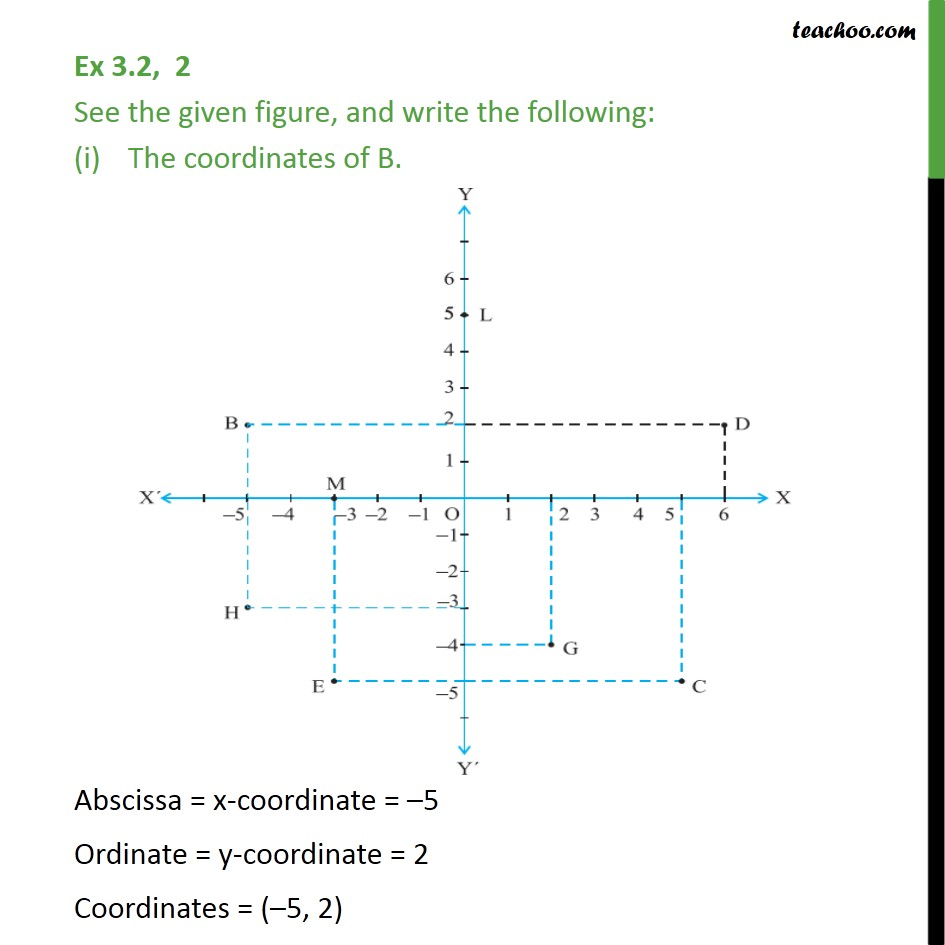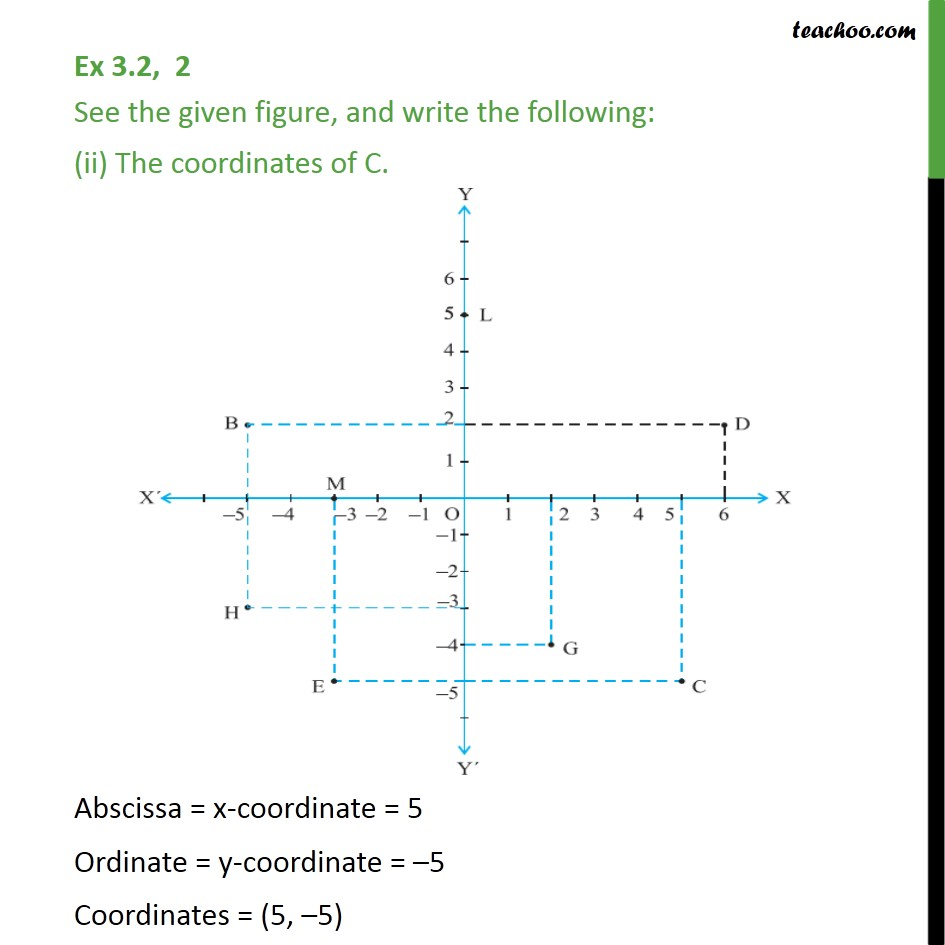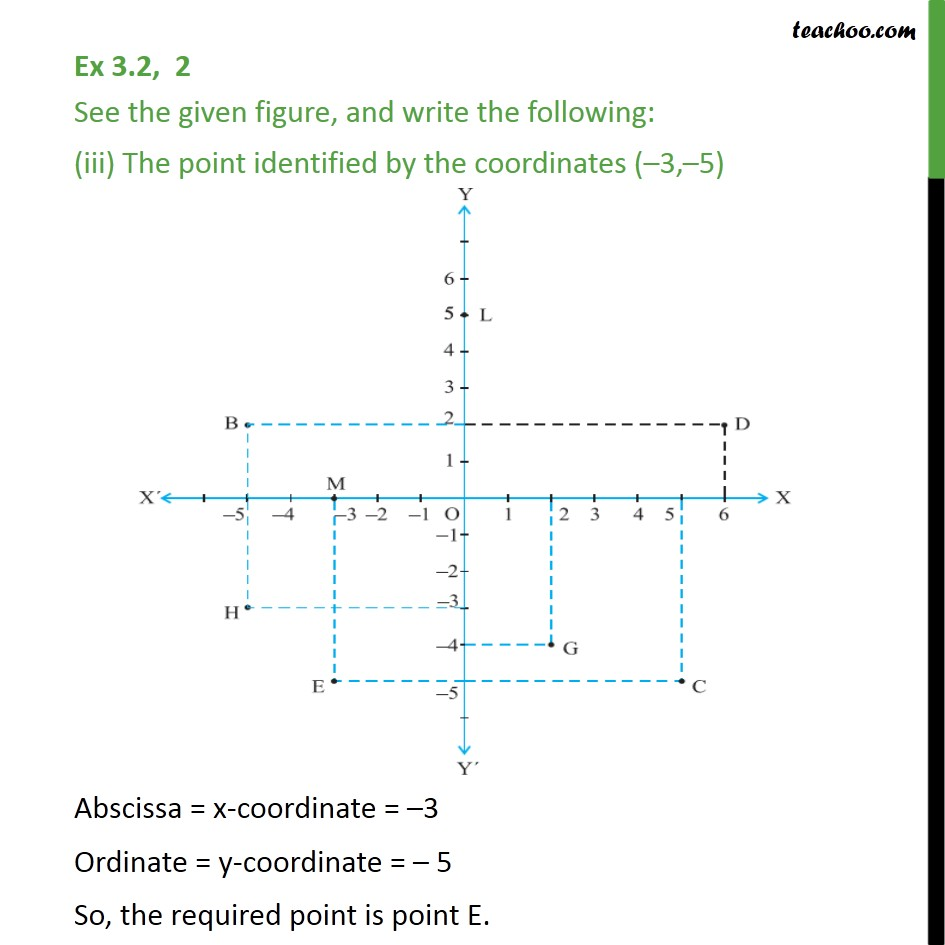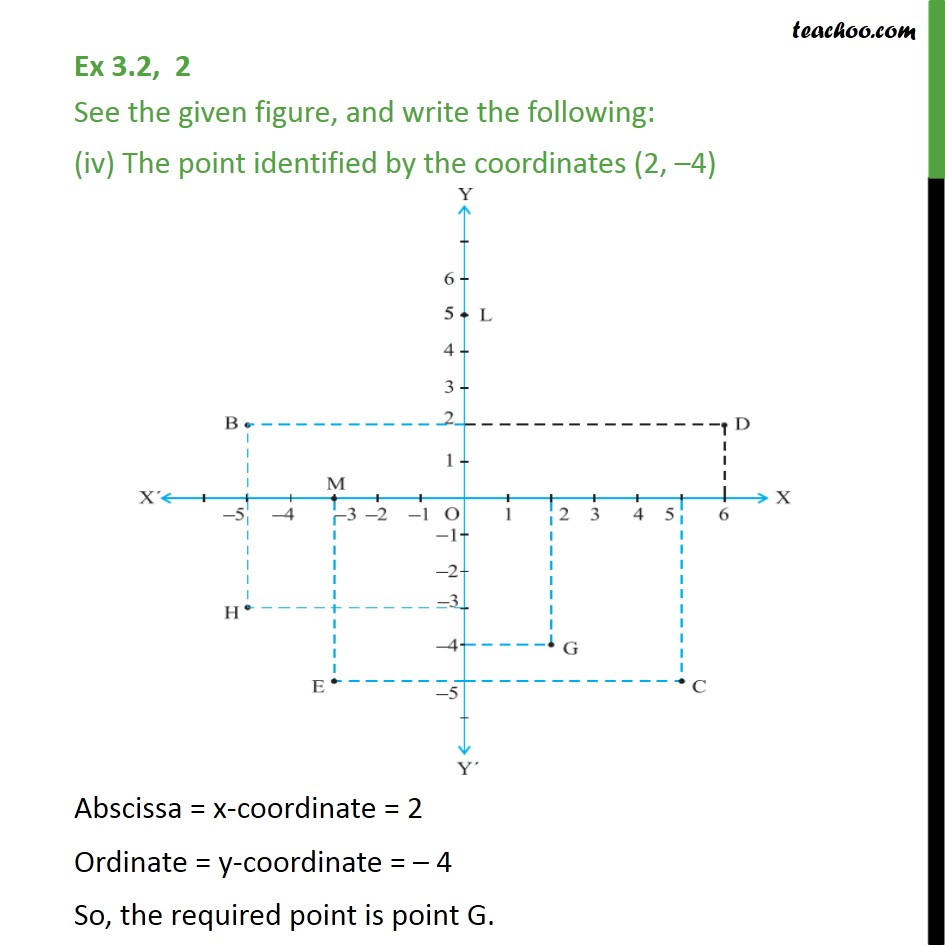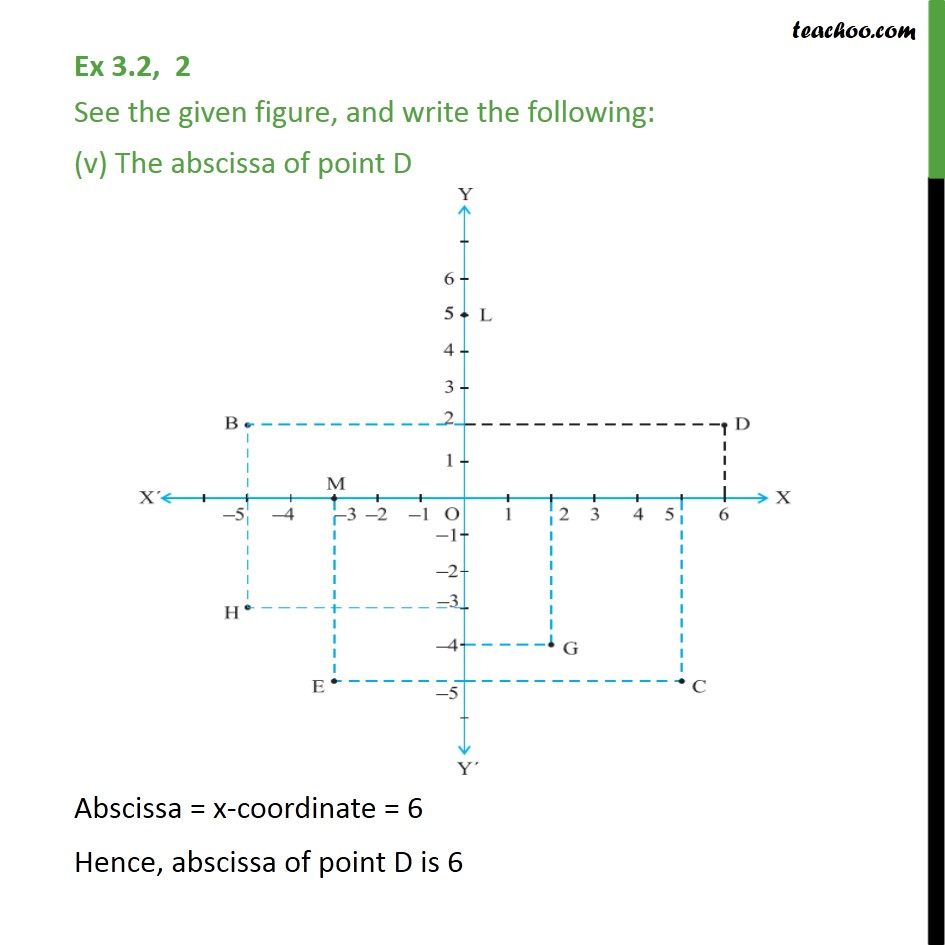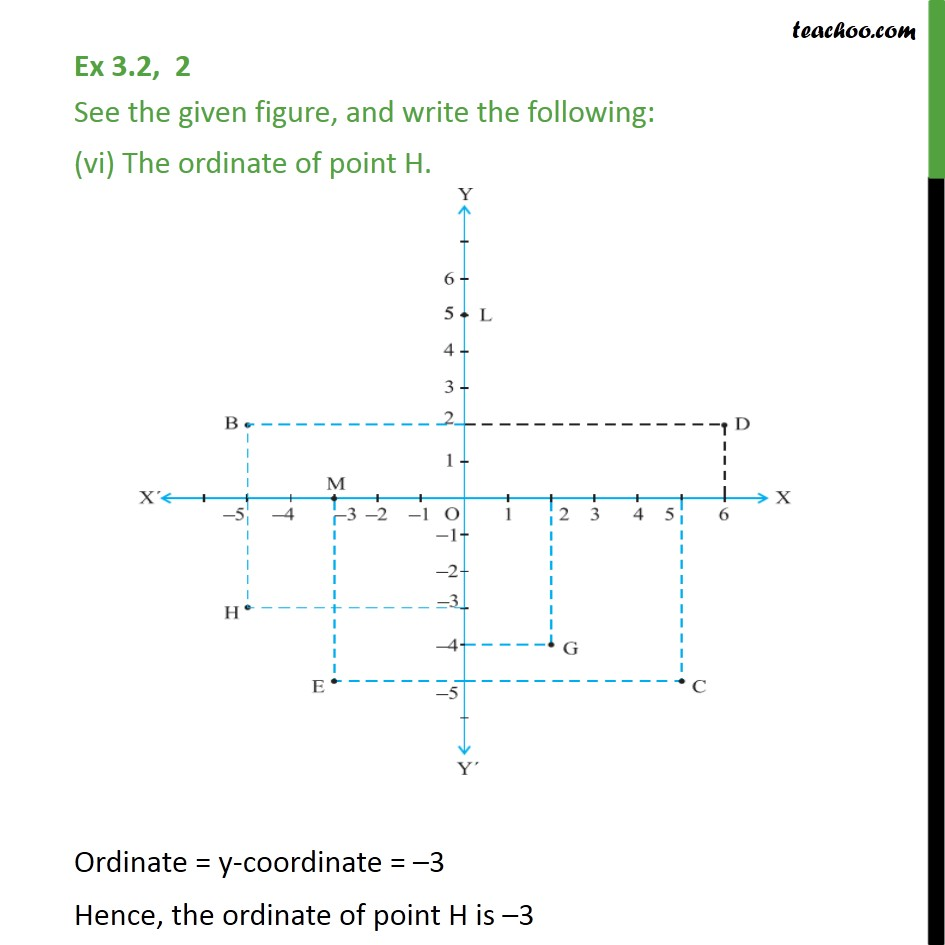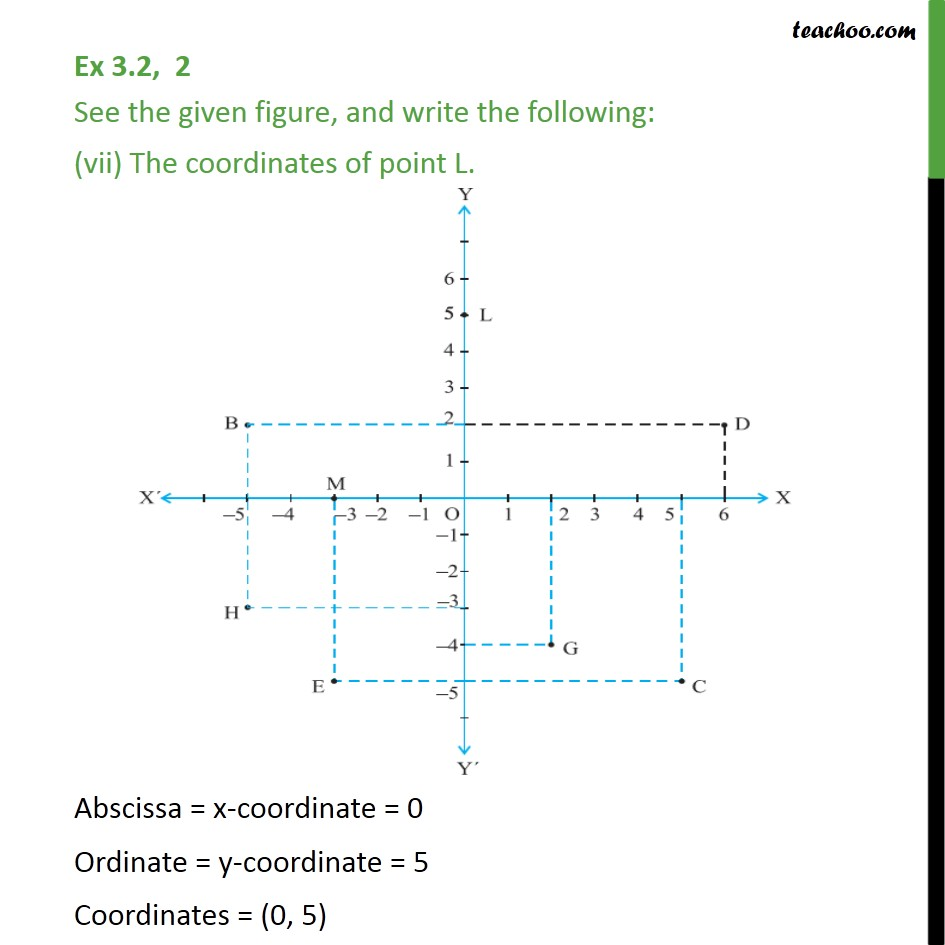1. Chapter 3 Class 9 Coordinate Geometry (Term 1)
2. Serial order wise
3. Ex 3.2

Transcript

Ex 3.2, 2 See the given figure, and write the following: The coordinates of B. Abscissa = x-coordinate = 5 Ordinate = y-coordinate = 2 Coordinates = ( 5, 2) Ex 3.2, 2 See the given figure, and write the following: (ii) The coordinates of C. Abscissa = x-coordinate = 5 Ordinate = y-coordinate = 5 Coordinates = (5, 5) Ex 3.2, 2 See the given figure, and write the following: (iii) The point identified by the coordinates ( 3, 5) Abscissa = x-coordinate = 3 Ordinate = y-coordinate = 5 So, the required point is point E. Ex 3.2, 2 See the given figure, and write the following: (iv) The point identified by the coordinates (2, 4) Abscissa = x-coordinate = 2 Ordinate = y-coordinate = 4 So, the required point is point G. Ex 3.2, 2 See the given figure, and write the following: (v) The abscissa of point D Abscissa = x-coordinate = 6 Hence, abscissa of point D is 6 Ex 3.2, 2 See the given figure, and write the following: (vi) The ordinate of point H. Ordinate = y-coordinate = 3 Hence, the ordinate of point H is 3 Ex 3.2, 2 See the given figure, and write the following: (vii) The coordinates of point L. Abscissa = x-coordinate = 0 Ordinate = y-coordinate = 5 Coordinates = (0, 5) Ex 3.2, 2 See the given figure, and write the following: (viii) The coordinates of point M. Abscissa = x-coordinate = 3 Ordinate = y-coordinate = 0 Coordinates = ( 3, 0)

Ex 3.2